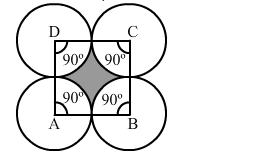# Four equal circles are described about the four corners of a square so that each touches two of the others, as shown in the figure.`
Question:

Four equal circles are described about the four corners of a square so that each touches two of the others, as shown in the figure. Find the area of the shaded region, if each side of the square measures 14 cm.Solution:

Side of the square = 14 cm

Radius of the circle $=\frac{14}{2}=7 \mathrm{~cm}$

Area of the quadrant of one circle $=\frac{1}{4} \pi r^{2}$

$=\frac{1}{4} \times \frac{22}{7} \times 7 \times 7$

$=38.5 \mathrm{~cm}^{2}$

Area of the quadrants of four circles $=38.5 \times 4=154 \mathrm{~cm}^{2}$

Now,

Area of the square $=(\text { Side })^{2}$

$=14^{2}$

$=196 \mathrm{~cm}^{2}$

Area of the shaded region = Area of the square - Area of the quadrants of four circles

$=196-154$

$=42 \mathrm{~cm}^{2}$